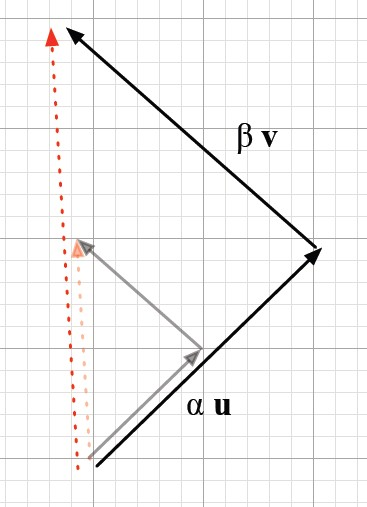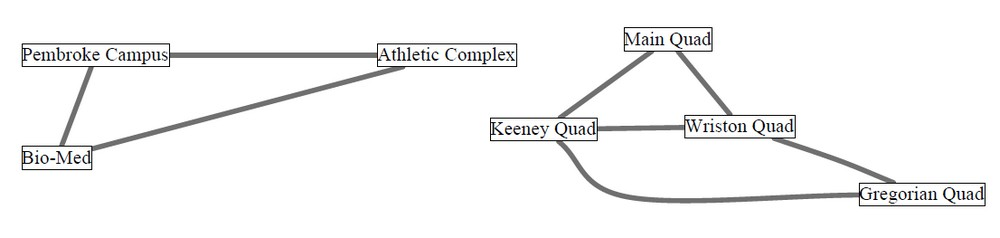# Linear Algebra - Linear Dependency

Vectors v1, . . . , vn are linearly dependent if the zero vector can be written as a nontrivial linear combination of the vectors: $$0 = \alpha_1 v_1 + \dots + \alpha_n v_n$$

In this case, we refer to the linear combination as a linear dependency in v1, . . . , vn. On the other hand, if the only linear combination that equals the zero vector is the trivial linear combination, we say v1, . . . , vn are linearly independent.

## Example

The vectors [1, 0, 0], [0, 2, 0], and [2, 4, 0] are linearly dependent, as shown by the following equation: $$2 [1, 0, 0] + 2 [0, 2, 0] - 1 [2, 4, 0] = [0, 0, 0]$$ Therefore: $2 [1, 0, 0] + 2 [0, 2, 0] - 1 [2, 4, 0]$ is a linear dependency in $[1, 0, 0], [0, 2, 0], [2, 4, 0]$ .

## When

When a list of vector $S = [v_0, \dots , v_n]$ are:

• not lineary independant:

$\begin{array}{lll} \href{dimension}{\text{dim }} \href{span}{\text{Span }} \{v_0, \dots , v_n\} < n + 1 \\ \href{rank}{rank} (v_0, \dots , v_n) < len(v_0, \dots , v_n) \end{array}$

• are lineary independant:

$\begin{array}{ll} \href{dimension}{\text{dim }} \href{span}{\text{Span }} \{v_0, \dots , v_n\} = n + 1 \\ \href{rank}{rank}(v_0, \dots , v_n) = len(v_0, \dots , v_n) \end{array}$

## Computation

def is_independent(L):
'''
input: a list L of vectors (using vec class)
output: True if the vectors form a linearly independent list.
'''
for i in range(len(L)):
if isABasis(L, i):
return True
return False


## How to know if vectors are linearly independent

### Easy

The vectors [1, 0, 0], [0, 2, 0], and [0, 0, 4] are linearly independent.

Since each vector has a nonzero entry where the others have zeroes.

Consider any linear combination $$\alpha_1 [1, 0, 0] + \alpha_1 [0, 2, 0] + \alpha_1 [0, 0, 4]$$ This equals to $$[\alpha_1, 2\alpha_2, 4\alpha_3]$$

If this is the zero vector, it must be that $\alpha_1 = \alpha_2 = \alpha_3 = 0$

That is, the linear combination is Linear Algebra - Linear combination.

Thus, the only linear combination that equals the zero vector is the trivial linear combination.

### Difficult

By linear-combinations definition, $v_1, \dots , v_n$ are linearly dependent iff there is a nonzero vector $\begin{bmatrix} \alpha_1 \\ \vdots \\ \alpha_n \end{bmatrix}$ such that $\begin{bmatrix} \begin{array}{r|r|} & & \\ v_1 & \dots & v_n \\ & & \end{array} \end{bmatrix} \begin{bmatrix} \alpha_1 \\ \vdots \\ \alpha_n \end{bmatrix} = 0$

Therefore, v1, . . . , vn are linearly dependent iff the Linear Algebra - Matrix of the matrix is nontrivial.

This shows that the question:

• How can we tell if vectors v1, . . . , vn are linearly dependent?

is the same as a question we asked earlier:

• How can we tell if the null space of a matrix is trivial?

is the same as :

• How can we tell if the solution set of a homogeneous linear system is trivial?

is the same as:

• How can we tell if a solution u1 to a linear system is the only solution?

Answering these questions requires an algorithm. Computational Problem: Testing linear dependence

• input: a list [v1, . . . , vn] of vectors
• output: DEPENDENDENT if the vectors are linearly dependent, and INDEPENDENT otherwise.

### if a subset of S form a cycle

We can get the zero vector by adding together vectors corresponding to edges that form a cycle: in such a sum, for each entry x, there are exactly two vectors having 1’s in position x.

Example: the vectors corresponding to {Main, Wriston},{Main, Keeney} {Keeney, Wriston } sum to the zero vector.

Therefore if a subset of S form a cycle then S is linearly dependent.

Example: The vectors corresponding to {Main, Keeney}, {Main, Wriston}, {Keeney, Wriston }, {Wriston, Gregorian} are linearly dependent because these edges include a cycle.

The zero vector is equal to the nontrivial linear combination:

Pembroke Athletic Bio-Med Main Keeney Wriston Gregorian
1 * 1 1
+ 1 * 1 1
+ 1 * 1 1
+ 0 * 1 1

### a set of edges contains no cycle

On the other hand, if a set of edges contains no cycle (i.e. is a forest) then the corresponding set of vectors is linearly independent.

## Algorithm

If they successfully finish, the Grow algorithm and the Shrink algorithm each find a set of vectors spanning the vector space V. In each case, the set of vectors found is linearly independent.

### Grow-Algorithm Corollary

Analyzing the Grow algorithm

def Grow(V)
S = 0;
repeat while possible:
find a vector v in V that is not in Span S, and put it in S.


Grow-Algorithm Corollary: The vectors obtained by the Grow algorithm are linearly independent.

In graphs, this means that the solution obtained by the Grow algorithm has no cycles (is a forest).

The Grow-Algorithm Corollary implies that, if the Grow algorithm terminates, the set of vectors it has selected is a basis for the vector space V.

### Shrink-Algorithm Corollary

def Shrink(V)
S = some finite set of vectors that spans V
repeat while possible:
find a vector v in S such that Span (S − {v}) = V, and remove v from S.


This algorithm use the Linear Algebra - Vector Space (set of vector).

Shrink-Algorithm Corollary: The vectors obtained by the Shrink algorithm are linearly independent.

In graphs, this means that the Shrink algorithm outputs a solution that is a forest.

The Shrink-Algorithm Corollary implies that, if we can run the Shrink algorithm starting with a finite set of vectors that spans V, upon termination it will have selected a basis for V.

Discover MoreLinear Algebra - Orthogonality (Perpendicular)

Orthogonal is linear-algebra-ese for perpendicular. The squared length of the “hypotenuse” (the vector u + v) is Thus following the Pythagorean Theorem u and v are orthogonal when then:...Linear Algebra - (Direct Sum | Union) of vector spaces

Let U and V be two vector spaces consisting of D-vectors over a field F. Definition: If U and V share only the zero vector then we define the direct sum of U and V to be the set: written: That is, ...Linear Algebra - (Gaussian|Common) Elimination

Method illustrated in Chapter Eight of a Chinese text, The Nine Chapters on the Mathematical Art, that was written roughly two thousand years ago. Rediscovered in Europe by Isaac Newton (England) and Michel...Linear Algebra - Basis of a Vector Space

A basis for vector space V is a linearly independent set of generators for V. Thus a set S of vectors of V is a basis for V if S satisfies two properties: Property B1 (Spanning) Span S = V, and Property...Linear Algebra - Graph

graph in linear algebra Formulating GF(2) vector: subset {Pembroke, Main, Gregorian} is represented by: Pembroke Athletic Bio-Med Main Keeney Wriston Gregorian 1 1 1 Edge Representation...Linear Algebra - Matrix

The Traditional notion of a matrix is: a two-dimensional array a rectangular table of known or unknown numbers One simple role for a matrix: packing together a bunch of columns or rows Matrix...Linear Algebra - Null Space of a (Matrix|Vector Space)

Null space of a matrix A (Written Null A) is: The Null space of a matrix is a basis for the solution set of a homogeneous linear system that can then be described as a homogeneous matrix equation. A...Linear Algebra - Rank

in linear algebra The rank of a set S of vectors is the dimension of Span S written: rank S dim Any set of D-vectors has rank at most |D|. If rank(S) = len(S) then the vectors are linearly dependent...Linear Algebra - Span of a Vector Space

The set of all linear combinations of some vectors v1,...,vn is called the span of these vectors and contains always the origin. Example: Let V = Span {[0, 0, 1], [2, 0, 1], [4, 1, 2]}. A vector belongs...